# Reflecting Shapes

Reflecting Shapes• Reflecting a shape means to flip the shape over a mirror line.
• The mirror line is shown as a dashed line.
• The shape is now facing the opposite direction to before.
• Every point on the shape is as far away from the mirror line as it was before.
• To reflect a shape we move the corners and then join them together at the end.
• The reflected shape image is the same size and shape as the original object.

The corners of a reflected shape are the same distance away from the mirror line.

The shape image is now facing the opposite direction to the original object.• To reflect the arrow in the mirror line we first mark the corners.
• We count how many squares each point is from the mirror line.
• The reflected point will be the same distance away from the mirror line but on the other side of the line.
• The tip of the arrow is 4 squares to the left of the line so its reflection will be 4 squares right of the line.
• The end of the arrow is 1 square left of the line so its reflection will be one square right of the line.
• Once all of the corners have been reflected, we connect them to complete our reflected image.
• The original object was pointing left but the reflected image is pointing right.# Reflecting Shapes

## What does Reflecting a Shape Mean?

Reflecting a shape simply means to flip it over a mirror line. Each point in the shape is moved to the other side of the mirror line but remains the same distance away from the line.

The reflected image will now be facing in the opposite direction to the original object.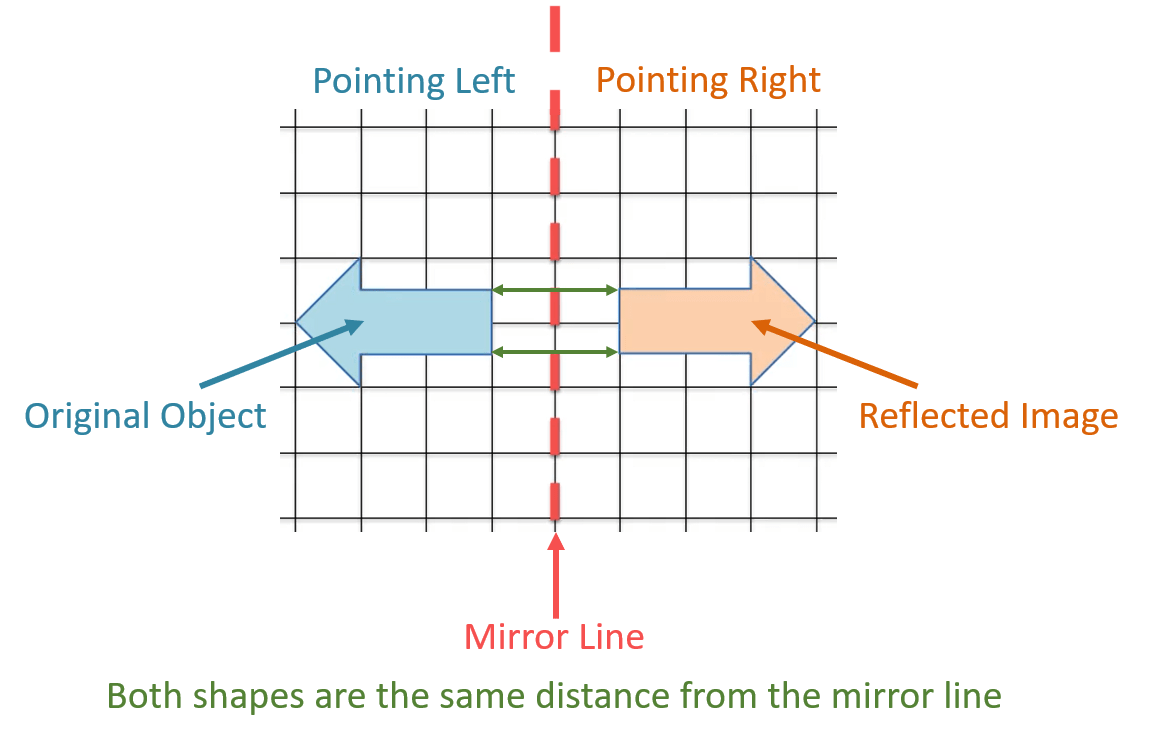The mirror line is the line that the shape is reflected in. It also called a line of reflection.

We can see in this example above that the arrow is pointing left to begin with. After it has been reflected in the mirror line, it now points right.

The end of the arrow is still one square away from the mirror line and the tip of each arrow is 4 squares away from the mirror line.After reflecting a shape, each point on the shape is the same distance away from the line of reflection but on the other side of the mirror line.

## How to Reflect a Shape in a Mirror Line

To reflect a shape in a mirror line use the following steps:

1. Mark the corners of the shape.
2. Place a ruler on one of these corners and on the mirror line so that the ruler is at right angles to the mirror line.
3. Measure how far away the corner is from the mirror line.
4. Without moving the ruler, measure this same distance from the mirror line but on the other side of the line.
5. This is the position of the reflected corner.
6. Repeat this for each corner of the shape.
7. Join the reflected corners together to create the reflected image of the shape.

Here is an example of reflecting a triangle in a line or reflection.

The first step is to mark each of the corners of the triangle.

We then use a ruler to measure how far away each corner is from the mirror line. When reflecting shapes on a grid, we can simply count the number of squares each corner is away from the mirror line.The corner nearest to the mirror line is 1 square to the left of the line.

Its reflection is 1 square to the right of the mirror line.

The other two corners of the triangle are 3 squares to the left of the mirror line and so, their reflection will be 3 squares to the right of the mirror line.

Once all corners have been reflected, the final step is to connect them with a ruler.

The original shape is called the object and the reflected shape is called the image.

Here is another example of reflecting a shape on a grid in a vertical mirror line.

The first step is to mark the corners of the trapezium.

We count how far away from the mirror line each point is and reflect it the same distance to the other side of the line.The two corners nearest to the mirror line are 2 squares to the left of the line. Their reflection will be 2 squares to the right of the line.

The other two corners furthest from the mirror line are both 4 squares left of the line. So their reflection will be 4 squares to the right of the line.

The reflected image is the same size and shape as the original object. It is just facing in the opposite direction.

We will now look at some examples of reflecting a shape in a horizontal mirror line.

Here we have a parallelogram.

The first step is to mark all of the corners of the parallelogram.

The second step is to count how far each corner is away from the mirror line. This time the points are above the mirror line and so we count how far above the mirror line each point is.

The reflected points will go below the line.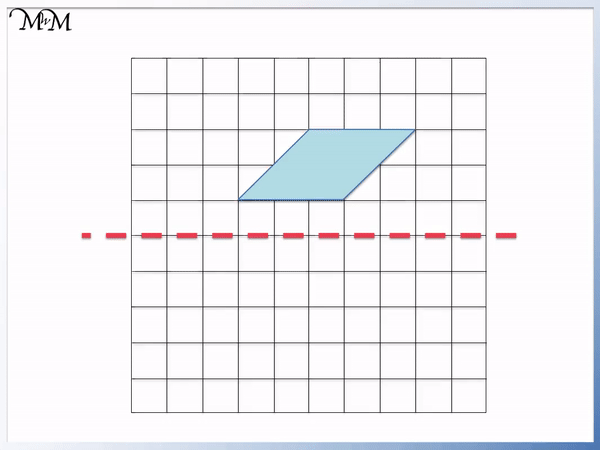The two corners of the shape nearest to the line of reflection are 1 square above the line.

Their reflection is 1 square below the line.

The two corners of the shape furthest from the line of reflection are 3 squares above the line.

Their reflection is 3 squares below the line.

We can see that the reflected image is the same size and shape as the original object.

Here is another example of reflecting a shape on a grid in a horizontal mirror line.

We can see that this shape will have a lot of corners. If this is the case it might be easier to reflect part of the shape and then draw the rest of it in afterwards.

Below we have a cross and so, we will reflect the central square of the cross and draw the rest of it in afterwards.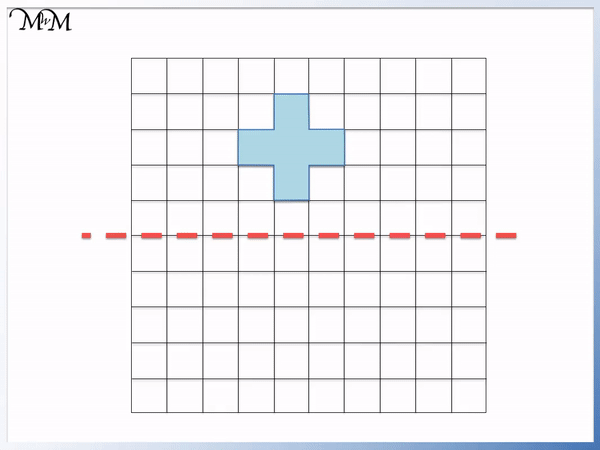The central square of the cross is 3 squares above the mirror line.

We can reflect the central square to 3 squares below the mirror line.

Now we can draw the rest of the cross.

The central square has a square next to each of its sides. We can simply add a square next to each side of this central square to complete our cross.

This method is easier than copying every corner across.

## Teaching Reflections in a Mirror Line

When first teaching reflections, it is helpful to have a small mirror to show how the reflected shape will look.

It is most helpful to do this with shapes such as arrows or triangles that are not symmetrical. That way, we can show how the shape has been reflected and how its orientation has changed. Shapes like arrows or triangles can be seen to be pointing in the opposite direction to before. If we use rectangles and squares, they will look the same because they are symmetric and we will be unable to see this effect.

We can also show reflections by folding paper.

We can use paint to colour in the inside of the original shape. Whilst the paint is still wet, we can fold the paper down the mirror line and press the two sides together.

The wet paint will leave the reflection of the shape on the other side of the mirror line when you open the paper up.

It can be helpful to look at lines of symmetry in everyday objects or photographs, which can act in a similar way to lines of reflection.

Below we have a picture of the Taj Mahal, with a mirror line down its centre.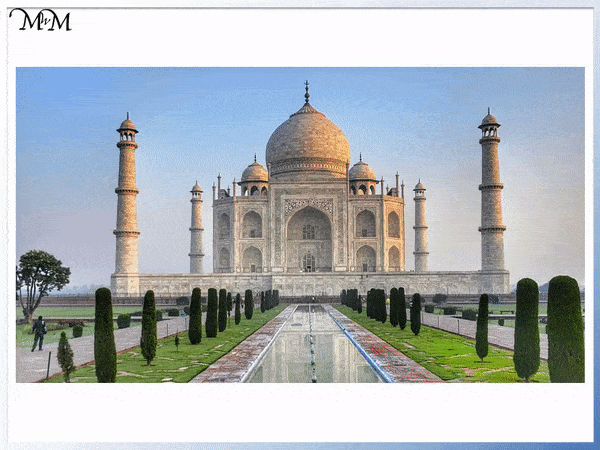We can see that each tower is the same distance away from the mirror line on each side.

We can also look for reflections in mirrors or reflections in water.

Below is a picture of some city buildings reflected in water.We can see that the reflected image is the same distance below the surface of the water as the original object.Now try our lesson on Translating Shapes where we learn how to translate different shapes.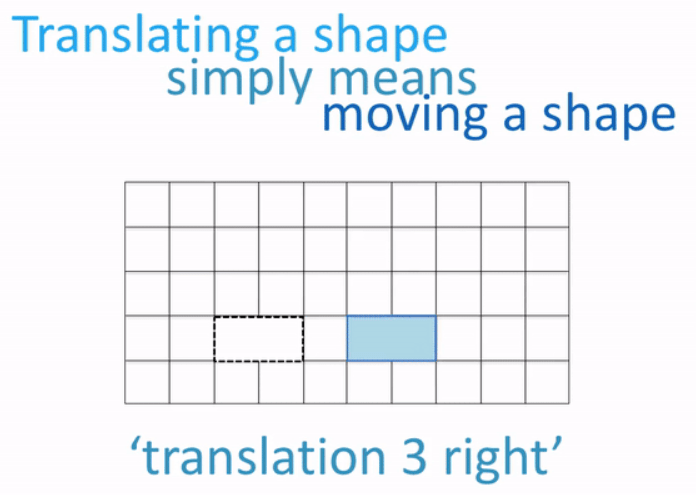error: Content is protected !!